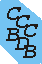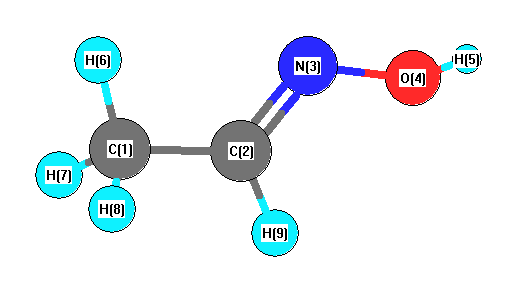Computational Chemistry Comparison and Benchmark DataBase Release 22 (May 2022) Standard Reference Database 101 National Institute of Standards and Technology Home All data for one species Geometry Experimental Calculated Comparisons Bad Calculations Tutorials and Explanations Vibrations Experimental Calculated Scale factors Reactions Entropies Ions List Ions Energy Electron Affinity Proton Affinity Ionization changes point group Experimental One molecule all properties One property a few molecules Geometry Vibrations Energy Electrostatics Reference Data Calculated Energy Optimized Reaction Internal Rotation Orbital Nuclear repulsion energy Correlation Ion Excited State Basis Set Extrapolation Geometry Vibrations Frequencies Zero point energy (ZPE) Scale Factors Bad Calculations Electrostatics Charges Dipole Quadrupole Polarizability Spin Entropy and Heat Capacity Reaction Lookup by property Comparisons Geometry Vibrations Energy Entropy Electrostatics Ion Resources Info on Results Calculations Done Basis functions used I/O files Glossary Conversion Forms Links NIST Links External links Thermochemistry Tutorials Vibrations Entropy Energy Electrostatics Geometry Cost Bad Calculations FAQ Help Units Choose Units Explanations Credits Just show me Summary Using List Recent molecules Molecules Geometry Vibrations Energy Similar molecules Ions, Dipoles, etc. Index of CCCBDB Feedback You are here: Home > Geometry > Experimental > Experimental Geometries OR Experimental > Geometry > Experimental Geometries

# Listing of experimental geometry data for CH3CHNOH (Acetaldoxime)

## Rotational Constants (cm-1)

See section I.F.4 to change rotational constant units
A B C
1.48814 0.14136 0.13255
0.57920 0.22212 0.16415
Rotational Constants from 1969Rog/Sch:397
Calculated rotational constants for CH3CHNOH (Acetaldoxime).

## Internal coordinates

(distances (r) in Å) (angles (a) in degrees) (dihedrals (d) in degrees)
Description Value Connectivity Reference Comment
Atom 1 Atom 2 Atom 3 Atom 4
rCN 1.276 2 3 1969Rog/Sch:397 !assumed
rNO 1.408 3 4 1969Rog/Sch:397 !assumed
rOH 0.956 4 5 1969Rog/Sch:397 !assumed
rCH 1.085 2 9 1969Rog/Sch:397 !assumed
rCH 1.100 1 6 1969Rog/Sch:397 !assumed
rCC 1.550 1 2 1969Rog/Sch:397 !assumed
aHON 103 3 4 5 1969Rog/Sch:397 !assumed
aCNO 110 2 3 4 1969Rog/Sch:397 !assumed
aHCN 122 3 2 9 1969Rog/Sch:397 !assumed
aCCN 116 1 2 3 1969Rog/Sch:397 !assumed
aHCH 109.4 6 1 7 1969Rog/Sch:397 !assumed## Bond descriptions

Examples: C-C single bond, C=C, double bond, C#C triple bond, C:C aromatic bond
Bond Type Count
C=N 1
N-O 1
H-O 1
H-C 4
C-C 1
Atom x (Å) y (Å) z (Å)

Atom - Atom Distances (Å)

Calculated geometries for CH3CHNOH (Acetaldoxime).
References